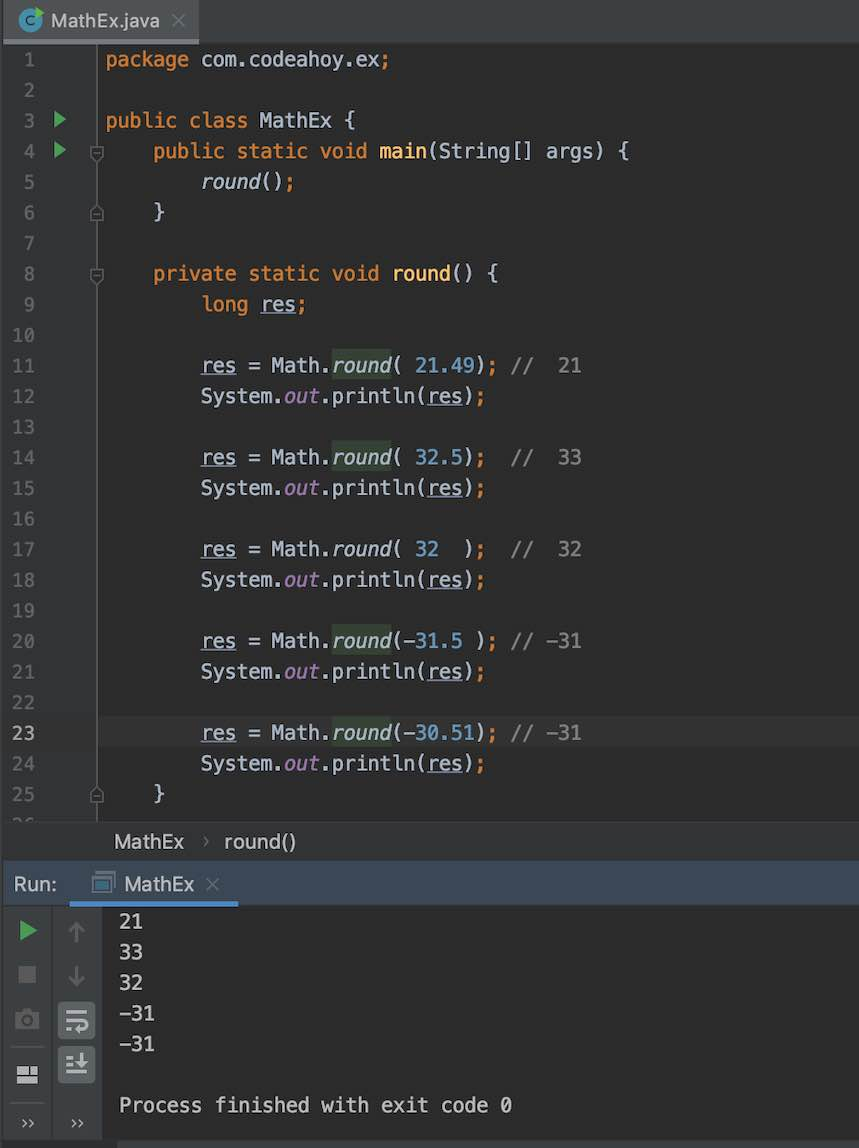# Java Math.round() with Examples

Oct 12, 2019 · 2 mins readThe java.lang.Math class that comes bundled with Java contains various methods for performing basic numeric operations such as the elementary exponential, logarithm, square root, and trigonometric functions.

Math.round(…) method is part of the Math class. It is used for rounding a decimal to the nearest integer. In mathematics, if the fractional part of the argument is greater than 0.5, it is rounded to the next highest integer. If it is less than 0.5, the argument is rounded to the next lowest integer.

### Math.round Method Signature

Math.round(…) method is overloaded. Here are the method signatures:

``````public static int round(float a)

public static long round(double a)
``````

Note just like all other methods on the Math class, Math.random(…) is a `static` method so you can call it directly on the Math class without needing an object. The overloaded methods take either `float` or `double` data type returning `int` or `long` respectively. This saves you from casting manually.

``````Math.round( 42.5);  //  43
``````

### Math.round Example

The following example illustrates how to use Math.round(…) method.

``````public class MathEx {
public static void main(String[] args) {
round();
}

private static void round() {
long res;

res = Math.round( 21.49); //  21
System.out.println(res);

res = Math.round( 32.5);  //  33
System.out.println(res);

res = Math.round( 32  );  //  32
System.out.println(res);

res = Math.round(-31.5 ); // -31
System.out.println(res);

res = Math.round(-30.51); // -31
System.out.println(res);
}
}
``````

Output

``````21
33
32
-31
-31
``````

Here’s a screenshot of the code above if you’re on mobile and having trouble reading the code above.### Special cases

• If the argument is NaN, the result is 0.
• If the argument is negative infinity or any value less than or equal to the value of Integer.MIN_VALUE, the result is equal to the value of Integer.MIN_VALUE.
• If the argument is positive infinity or any value greater than or equal to the value of Integer.MAX_VALUE, the result is equal to the value of Integer.MAX_VALUE.

If you need more detailed information, please see Javadocs. It’s worth noting that the `Math` class in Java contains several other useful methods for arithmetic, logarithms and trignometric operations.

#### You May Also Enjoy

If you like this post, please share using the buttons above. It will help CodeAhoy grow and add new content. Thank you!